# Six Basic Trigonometric Functions

Go back to  'Trigonometry'

In this section, we’ll briefly recapitulate the fundamentals of trigonometry, assuming that you’re already familiar with the material presented.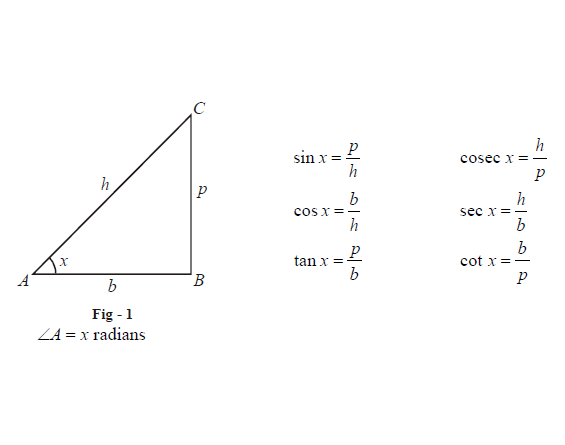$$(a)\;\;\; f (x) = sin\; x$$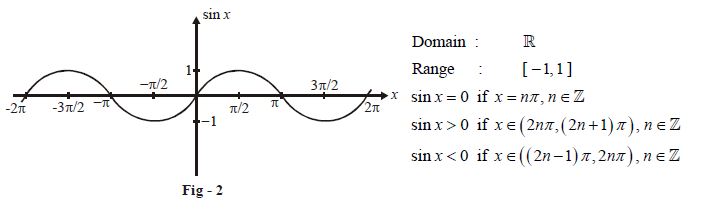$$(b)\;\;\; f (x) = cos\; x$$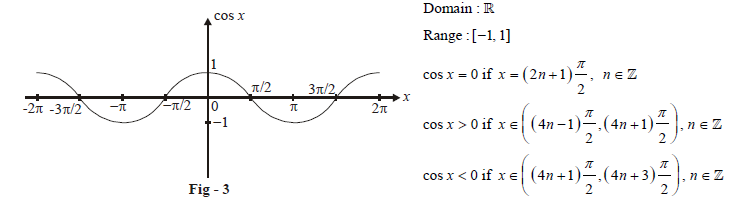$$(c)\;\;\; f (x) = tan \;x$$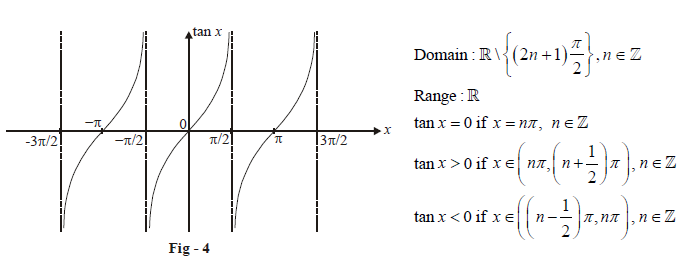$$(d)\;\;\; f (x) = cot\; x$$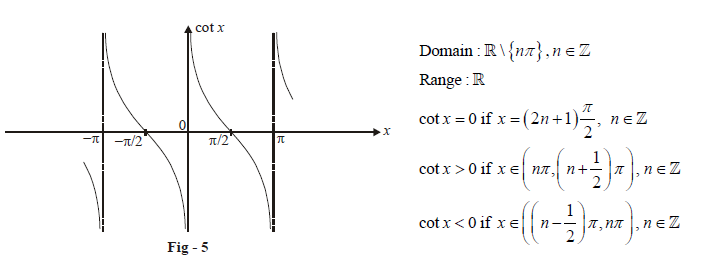$$(e)\;\;\; f (x) = sec\; x$$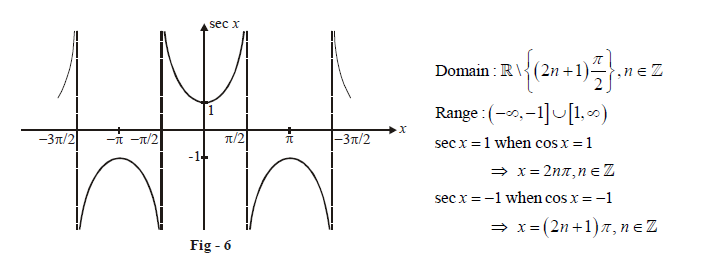$$(f)\;\;\; f (x) = cosec\; x$$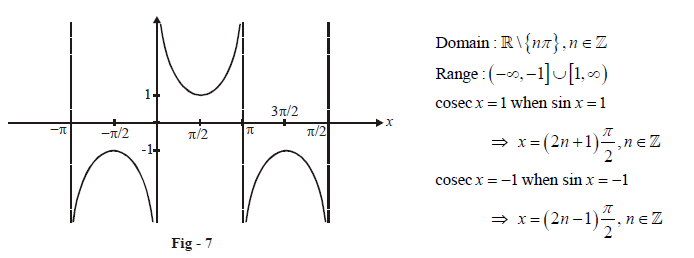It is important to be familiar with these six basic functions and their properties for further study of trigonometry.

Listed below are basic relations satisfied by these functions:
(A)

 $${\sin ^2}x + {\cos ^2}x = 1$$ $$1 + {\tan ^2}x = {\sec ^2}x$$ $$1 + {\cot ^2}x = {\text{cose}}{{\text{c}}^2}x$$

_____________________________________________________________________
(B)

 $$\sin \left( { - x} \right) = - \sin x$$ $$\cos \left( { - x} \right) = \cos x$$ $$\tan \left( { - x} \right) = - \tan x$$ $$\cot \left( { - x} \right) = - \cot x$$ $$\sec \left( { - x} \right) = \sec x$$ $${\text{cosec}}\left( { - x} \right) = - {\text{cosec}}\;x$$

____________________________________________________________________
(C)

 \begin{align}\sin \left( {\frac{\pi }{2} \mp x} \right) = {\text{cos}}\;x\end{align} $$\sin \left( {\pi - x} \right) = \sin \;x$$ $$\sin \left( {\pi + x} \right) = - \sin \;x$$ \begin{align}\cos \left( {\frac{\pi }{2} \mp x} \right) = \pm \sin \;x\end{align} $$\cos \left( {\pi - x} \right) = - \cos \;x$$ $$\cos \left( {\pi + x} \right) = - \cos \;x$$ \begin{align}\tan \left( {\frac{\pi }{2} \mp x} \right) = \pm \cot \;x\end{align} $$\tan \left( {\pi \pm x} \right) = \pm \tan \;x$$

The properties in (A) follow from the definitions of these functions, while those in (B) and (C) can be inferred from the graphs of these functions.